# FunctionsPage 1

#### WATCH ALL SLIDES

Slide 1Slide 2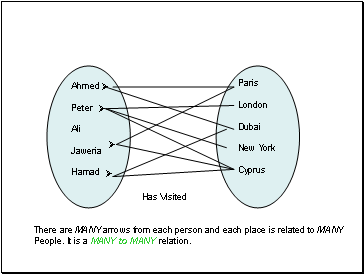Ahmed

Peter

Ali

Jaweria

Paris

London

Dubai

New York

Cyprus

Has Visited

There are MANY arrows from each person and each place is related to MANY

People. It is a MANY to MANY relation.

Slide 3Bilal

Peter

Salma

Alaa

George

Aziz

62

64

66

Person Has A Mass of Kg

In this case each person has only one mass, yet several people have the same

Mass. This is a MANY to ONE relationship

Slide 4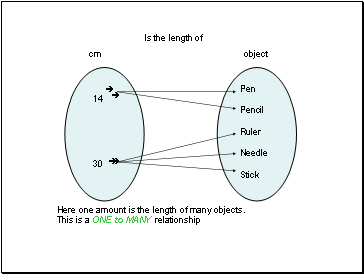Is the length of

14

30

Pen

Pencil

Ruler

Needle

Stick

cm

object

Here one amount is the length of many objects.

This is a ONE to MANY relationship

Slide 5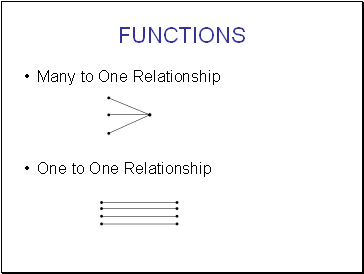## Functions

Many to One Relationship

One to One Relationship

Slide 6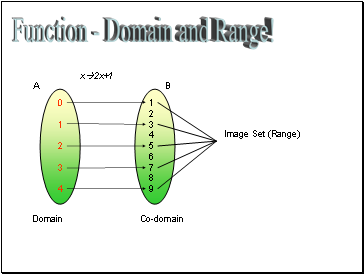## Function - Domain and Range!

Domain Co-domain

0

1

2

3

4

1

2

3

4

5

6

7

8

9

Image Set (Range)

x2x+1

A

B

Slide 7## Functions - Notation

The upper function is read as follows:-

‘Function f such that x is mapped onto x2+4

Slide 8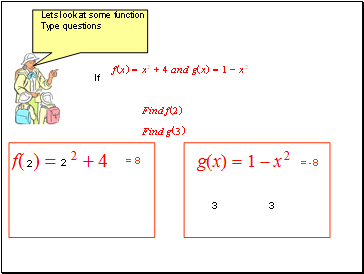If

2

2

= 8

3

3

= -8

Slide 9## Flow Diagrams

We can consider this as two simpler

functions illustrated as a flow diagram

Multiply by 3

Subtract 1

Multiply by 2

Square

Slide 10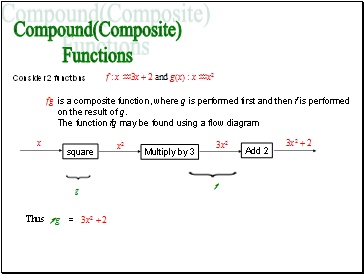## Compound(Composite)

Functions

Consider 2 functions

is a composite function, where g is performed first and then f is performed

on the result of g.

The function fg may be found using a flow diagram

square

Multiply by 3

Slide 11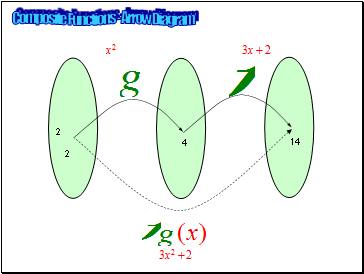2

4

14

2

Composite Functions - Arrow Diagram

Slide 12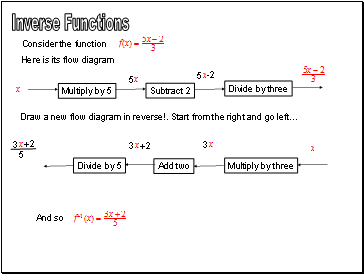Go to page:
1  2